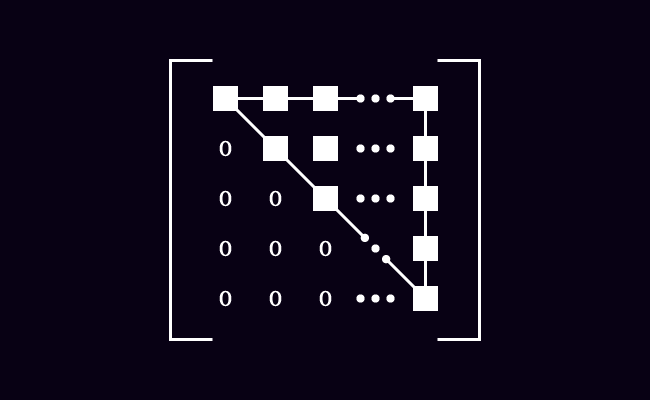# Upper Triangular matrix

## Definition

A square matrix in which all the entries below the main diagonal are zero, is called an upper triangular matrix.

### IntroductionFirstly, let’s know the meanings of the words “upper” and “triangular” before learning the concept of an upper triangular matrix and its internal structure.

1. The word “Upper” means situated above another part.
2. The word “Triangular” means shaped like a triangle.

In a special case, the elements below the leading diagonal are zero in a square matrix, the entries on the main diagonal and elements above the principal diagonal form a triangle shape. Hence, the square matrix is called an upper triangular matrix.

$U \,=\, \begin{bmatrix} e_{11} & e_{12} & e_{13} & \cdots & e_{1n}\\ 0 & e_{22} & e_{23} & \cdots & e_{2n}\\ 0 & 0 & e_{33} & \cdots & e_{3n}\\ \vdots & \vdots & \vdots & \ddots & \vdots\\ 0 & 0 & 0 & \cdots & e_{nn} \end{bmatrix}$

The matrix $U$ is basically a square matrix of order $n$. In this matrix, $e_{11}$, $e_{22}$, $e_{33}$, $e_{44}$ $\cdots$ $e_{nn}$ are the elements on the primary diagonal. The entries on the major diagonal and the elements above the main diagonal form a triangle shape but the entries below the leading diagonal are zeros. Therefore, the matrix $U$ is called an upper triangular matrix.

#### Condition

$U \,=\, {\begin{bmatrix} e_ij} \end{bmatrix}}_n \times n$

In an upper triangular matrix, the elements are zero if $i > j$ and they are elements under the main diagonal of the matrix but the remaining elements are non-zero elements and form a triangle shape.

In other words, $e_{ij} = 0$ if $i > j$.

#### Examples

Look at the following three matrices to understand the concept of upper triangular matrix.

$A \,=\, \begin{bmatrix} 2 & -4\\ 0 & 3 \end{bmatrix}$

The matrix $A$ is a square matrix of order $2$.

1. The entries $2$ and $3$ are elements on the main diagonal.
2. The entry above the principal diagonal is $-4$ but the entry below the primary diagonal is $0$.
3. The entries on the leading diagonal $2$ and $3$ and the entry above the major diagonal $-4$ form a triangle shape.

So, the matrix $A$ is called an upper triangular matrix.

$B \,=\, \begin{bmatrix} 1 & 5 & 6\\ 0 & 3 & 7\\ 0 & 0 & 4 \end{bmatrix}$

The matrix $B$ is a square matrix of order $3$.

1. The elements $1$, $3$ and $4$ are entries on the major diagonal.
2. The elements above the leading diagonal are $5$, $6$ and $7$ but the entries below the main diagonal are $0$.
3. The elements on the principal diagonal $1$, $3$ and $4$ and the entries above the primary diagonal form a triangle shape.

Hence, the matrix $B$ is called an upper triangular matrix.

$C = \begin{bmatrix} 4 & 2 & -3 & 9\\ 0 & -1 & 6 & -5\\ 0 & 0 & 8 & 3\\ 0 & 0 & 0 & 5\\ \end{bmatrix}$

The matrix $C$ is a square matrix of the order $4$.

1. The elements $4$, $-1$, $8$ and $5$ are the entries on the leading diagonal.
2. The entries above the primary diagonal are $2$, $-3$, $9$, $6$ and $-5$ but the elements below the main diagonal are $0$.
3. The elements on the major diagonal $4$, $-1$, $8$ and $5$ with the entries above the principal diagonal form a triangle shape.

Therefore, the matrix $C$ is called an upper triangular matrix.

Latest Math Topics
Jun 26, 2023
Jun 23, 2023

###### Math Questions

The math problems with solutions to learn how to solve a problem.

Learn solutions

Practice now

###### Math Videos

The math videos tutorials with visual graphics to learn every concept.

Watch now

###### Subscribe us

Get the latest math updates from the Math Doubts by subscribing us.